# K-邻近算法

## K最近邻算法

### K-邻近算法概述

K-近邻算法是几种基本的分类和回归算法之一。它的输入是实例的特征向量，对应于特征空间中的点，输出是实例的类别。

k-近邻算法（KNN），它的工作原理是：存在一个样本数据集合，也称作训练样本集，并且样本集中每个数据都存在标签，即我们知道样本集中每一数据与所属分类的对应关系。输入没有标签的新数据后，将新数据的每个特征与样本集中数据对应的特征进行比较，然后算法提取样本集中特征最相似数据（最近邻）的k个分类标签

### KNN的工作原理：

1. 计算分类样本与其他训练样本的距离； [距离测量]
2. 统计k个近邻【k值选择】
3. k个邻居中出现次数最多的标签就是样本的标签【分类决策】

#### k值选择

k值对结果有重要影响。如果k值太小，则整体训练的模型会变得复杂并且容易出现过拟合。 k值将涵盖大多数样本的特征，并且可能欠拟合。

### KNN算法步骤：

1. 加载和组织数据；
2. 计算待测试样本与各训练集数据的距离，并从小到大排序；
3. 确定并取出前k个值（即距离最小的k个值）；
4. 返回k个值中出现次数最多的标签；
5. 待测试的样本就是这一类。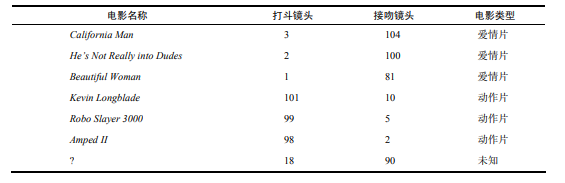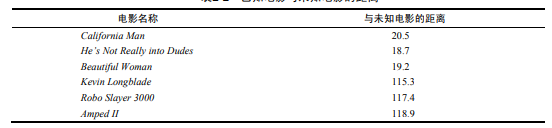### 算法代码实例

knn处理的数据类型有：数值型和标称型。

//将上面的表格数据处理
/*

*/
#导入必备包
import numpy as np
import math
#加载数据并整理数据
data=[[1,3,104,0],[2,2,100,0],[3,1,81,0],[4,101,10,1],[5,99,5,1],[6,98,2,1]]
data=np.array(data)
#求[?,18,90,?]

#求距离并排序
x=data[:,1:2]
y=data[:,2:3]
z=[18,90]
for i,j in zip(x,y):
distance=math.sqrt((z-i)**2+(z-j)**2)
test_distance.append(distance)
test_distance=[round(x,2) for x in test_distance]
print(test_distance)

[20.52, 18.87, 19.24, 115.28, 117.41, 118.93]
example=[]
for i,j in zip(data,target_distance):
x=np.append(i,j)
example.append(x)
example

[array([  1.  ,   3.  , 104.  ,   0.  ,  20.52]),
array([  2.  ,   2.  , 100.  ,   0.  ,  18.87]),
array([ 3.  ,  1.  , 81.  ,  0.  , 19.24]),
array([  4.  , 101.  ,  10.  ,   1.  , 115.28]),
array([  5.  ,  99.  ,   5.  ,   1.  , 117.41]),
array([  6.  ,  98.  ,   2.  ,   1.  , 118.93])]

#k取3时，标签全为0，第四位为标签，0为爱情片，故未知电影为爱情片。



python的sklearn库实现了knn算法（iris数据集）：

from sklearn.datasets import load_iris   #从数据集导入鸢尾花数据集
from sklearn.model_selection import train_test_split   #导入训练集和测试集分割函数
from sklearn.neighbors import KNeighborsClassifier   #导入knn分类器

#加载数据并整理
#分割数据集为训练集和测试集(.data为特征，.target为标签，test_size为测试集比重)
x_train,x_test,y_train,y_test=train_test_split(iris.data,iris.target,test_size=0.3)

#构造分类器
knn=KNeighborsClassifier(n_neighbors=3)  #n_neoghbors即为k

#训练模型
knn.fit(x_train,y_train)

#对测试集预测并对模型评分
score=knn.score(x_test,y_test)
print(score)

1.0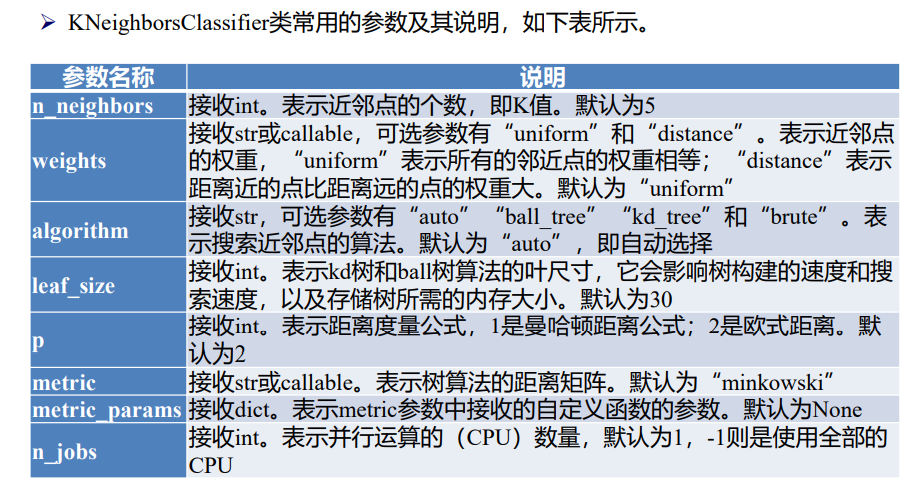KNeighborsClassifier对象也提供属性来操作模型：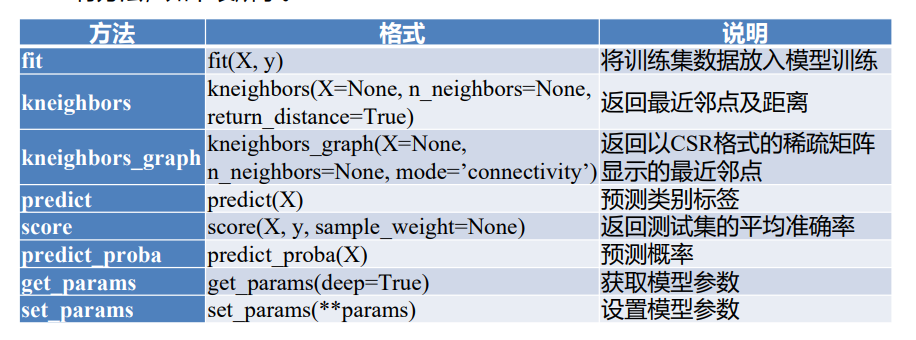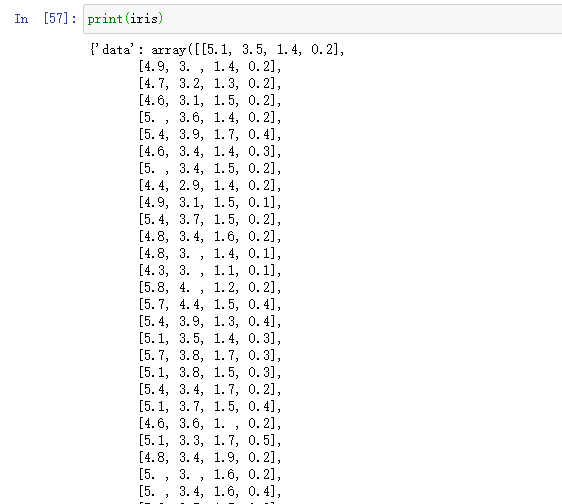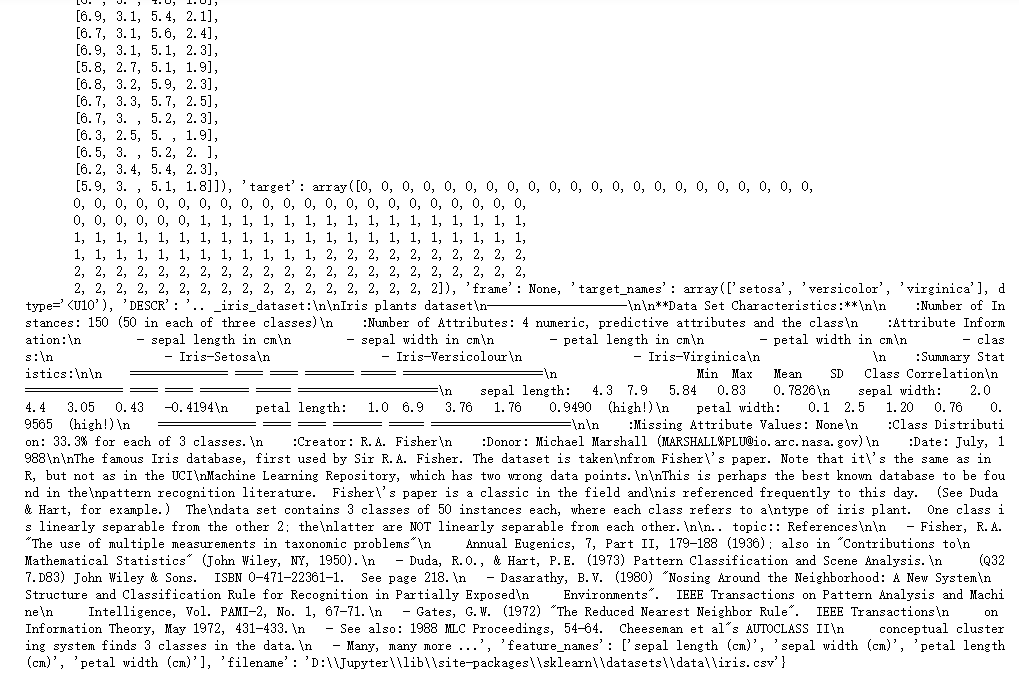// 数据集特征
print(len(iris.data))

150
print(len(iris.target))

150
print(iris.data[:5])

[[5.1 3.5 1.4 0.2]
[4.9 3.  1.4 0.2]
[4.7 3.2 1.3 0.2]
[4.6 3.1 1.5 0.2]
[5.  3.6 1.4 0.2]]
print(iris.target)

0

#可见特征和标签的长度一致，且对应，前5个的数据标签都为0.

#测试集的数据结构也要为同类型
a_data=[5,3.6,1.4,0.2]   a_target=?
#a_data是list而iris.data是numpy下的array,进行数据转换
a_data=np.array(a_data).reshape(1,-1)
knn.predict(a_data)

array()



import matplotlib.pyplot as plt
import numpy as np
def function(k):
x_train,x_test,y_train,y_test=train_test_split(iris.data,iris.target,test_size=0.3)
knn=KNeighborsClassifier(n_neighbors=k)  #n_neoghbors即为k
knn.fit(x_train,y_train)
score=knn.score(x_test,y_test)
return round(score.tolist(),2)

k=[1,2,3,4,5,10,20,60,100]
score_=[]
score_.append(function(1))
score_.append(function(2))
score_.append(function(3))
score_.append(function(4))
score_.append(function(5))
score_.append(function(10))
score_.append(function(20))
score_.append(function(60))
score_.append(function(100))
#print(score_)

plt.scatter(k,score_)
plt.show()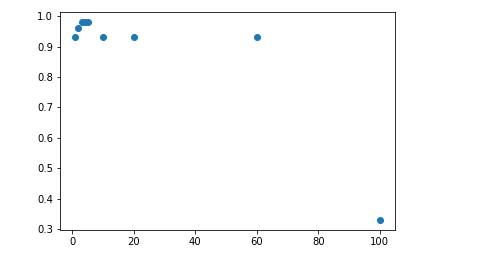. . .

## 相关推荐

#### 创建新模块后，swagger无法扫描新模块中的控制器接口。

框架默认情况下，只能扫描ruoyi-admin模块下的controller接口。如果需要扫描其它子模块中的swagger注解，那么需要调整一下swagger相关内容。 目录 1、前置条件 2、解决办法 1、前置条件 首先，我们要知道，默认情况下swag

#### zk 搭建maven工程，建立curator与zkserver的连接

创建客户端

#### 1726: 打印菱形

1726: 打印菱形 时间限制: 1 Sec 内存限制: 128 MB #include <iostream> using namespace std; //规律: 上三角形的行数=用户输入的值 // 下三角形的行数=用户输入的值-1 //打印上三角形

#### Unity与Android Studio交互之 ✨ 获取手机权限（存储、录音、相机等）

Unity通过Android Studio 获取手机权限（存储、录音、相机等） 文章目录 Unity通过Android Studio 获取手机权限（存储、录音、相机等） 常用权限 一、动态获取权限流程 二、使用步骤 1.检测当前是否已获取权限 2.检测当

#### Ctfhub - web -- SQL注入

SQL注入 1.整型注入 2.字符型注入 3.报错注入 4.布尔盲注 方法一：sqlmap 方法二：python脚本 1.整型注入 随便输入一个1，很明显这里已经给出了字段，不需要再order by了 1.数据库名和版本。这里发现是高版本，有inform

#### 软件设计师笔记系列（四）

-前言 随着技术的快速发展，软件已经成为我们日常生活中不可或缺的一部分。从智能手机应用到大型企业系统，软件都在为我们提供便利、增强效率和创造价值。然而，随之而来的是对软件质量的日益增长的关注。软件的质量不仅关乎其功能性和性能，还涉及其可靠性、使用性、可维

29天前
28天前
27天前
25天前
25天前
25天前
25天前
25天前
25天前
24天前

28天前
28天前
27天前
25天前
25天前
25天前
25天前
22天前
17天前